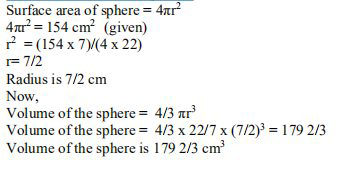# NCERT Solutions Class 9 Mathematics Solutions for Surface Areas and Volumes - Exercise 13.8 in Chapter 13 - Surface Areas and Volumes

Find the volume of a sphere whose surface area is 154 \mathrm{cm}^{2} . \text { (Assume } \left.\pi=22 / 7\right)

A sphere's volume is a measurement of how much space it can occupy. A three-dimensional shape with no edges or vertices is known as a sphere.

The surface area of a sphere is the area covered by the sphere's outer surface.

Let r be the radius of a sphere.Related Questions

Lido

Courses

Teachers

Book a Demo with us

Syllabus

Maths
CBSE
Maths
ICSE
Science
CBSE

Science
ICSE
English
CBSE
English
ICSE
Coding

Terms & Policies

Selina Question Bank

Maths
Physics
Biology

Allied Question Bank

Chemistry
Connect with us on social media!